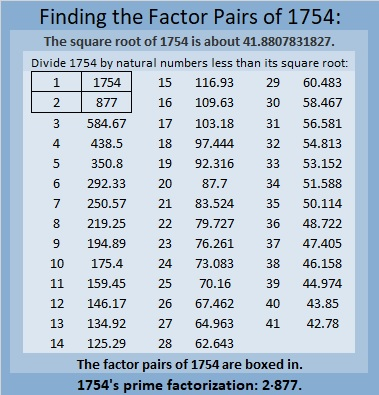# 1754 and a Level 2 Puzzle

### Today’s Puzzle:

Can you write each number from 1 to 12 in the first column and again in the top row so that the products of the numbers you write are the given clues?### Factors of 1754:

• 1754 is a composite number.
• Prime factorization: 1754 = 2 × 877.
• 1754 has no exponents greater than 1 in its prime factorization, so √1754 cannot be simplified.
• The exponents in the prime factorization are 1 and 1. Adding one to each exponent and multiplying we get (1 + 1)(1 + 1) = 2 × 2 = 4. Therefore 1754 has exactly 4 factors.
• The factors of 1754 are outlined with their factor pair partners in the graphic below.### More About the Number 1754:

1754 is the sum of two squares:
35² + 23² = 1754.

1754 is the hypotenuse of a Pythagorean triple:
696-1610-1754, calculated from 35² – 23², 2(35)(23), 35² + 23².
It is also 2 times (348-805-877).